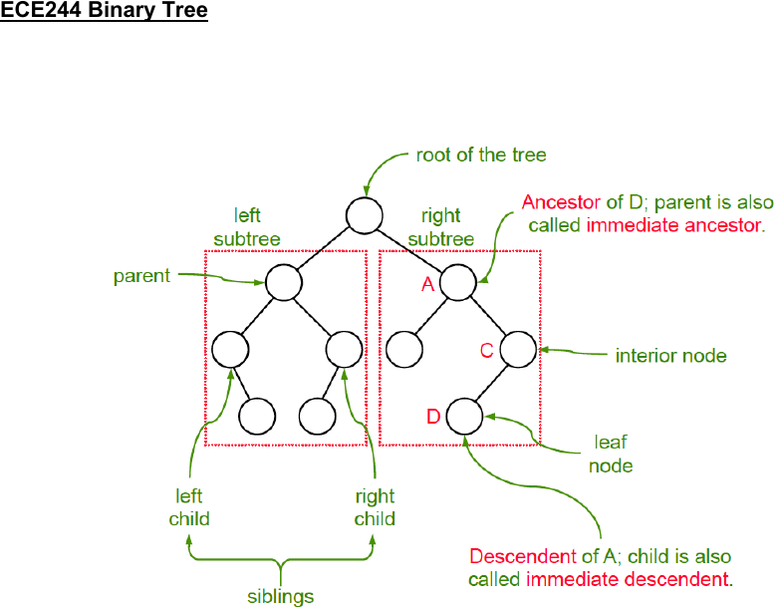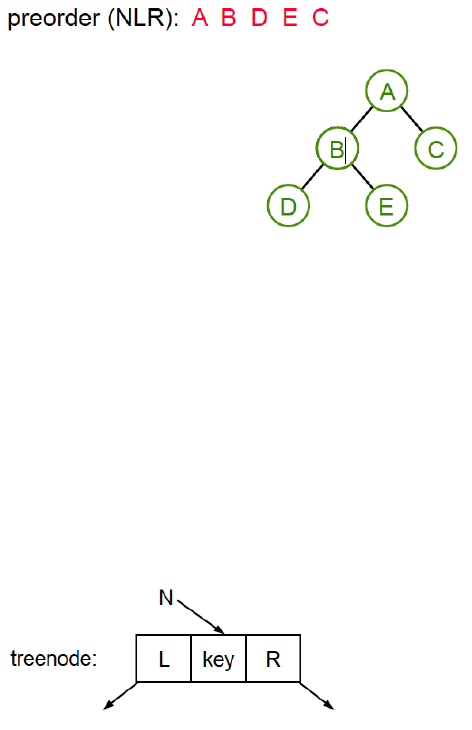Textbook Notes (280,000)
CA (170,000)
UTSG (10,000)
ECE (80)
Chapter 17

# ECE244H1 Chapter 17: ECE244 Binary Tree

Department
Electrical and Computer Engineering
Course Code
ECE244H1
Professor
Tarek Abdelrahman
Chapter
17

This preview shows pages 1-2. to view the full 7 pages of the document.ECE244 Binary Tree
What is a binary tree?
- Structure that is either empty or consists of one node connected to two disjoint
structure
- There are unique parents for each node in the tree except for root
- Length of path = numbers of node - 1
- Path between the root and each node in the tree is unique
What is Tree Traversals?
- Visiting each node once and only once
- There are 6 possibilities but only 3 are USEFUL
In-order traversal (LNR)
Traverse left → visit node → traverse right
Pre-order traversal (NLR)
Visit node → traverse left → traverse right
Post-order traversal (LRN)
Traverse left → traverse right → visit node
- Traverse left / traverse right means traverse left subtree or traverse right subtree in
the same order

Only pages 1-2 are available for preview. Some parts have been intentionally blurred.Pre-order traversal (NLR)
- Visit node → traverse left → traverse right
(visit the left subtree first and come back to the right subtree)
What’s a Binary Search Tree?
- Binary tree is sorted when using in-order traversal (LNR)
(left subtree -> node -> right subtree)
- Each node has a key
- key of any node is greater than the keys of the nodes in its left subtree
- key of any node is less than the keys of the nodes in its right subtree
- Order: L < N < R this is why binary tree is sorted using in-order traversal
(left subtree < node < right subtree)
< C++ Code >
treenode* searchBST(treenode* N, int skey){
if(N == NULL)
return NULL;
if(N->key == skey)
return N;
else if(N->key < skey) //must be in left subtree
return searchBST(N->R, skey);
else //must be in right subtree
return searchBST(N->L, skey);